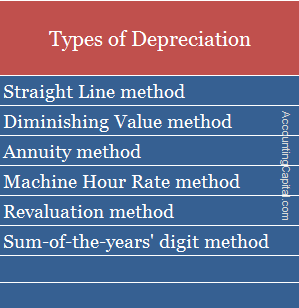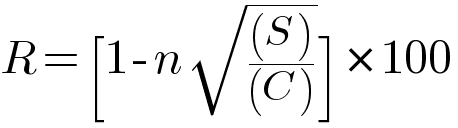# What is Depreciation and its Types?

## Depreciation

Depreciation is a reduction in the value of a tangible fixed asset due to normal usage, wear and tear, new technology, or unfavourable market conditions. Unlike amortization, which is applied to intangible assets, depreciation is applicable to tangible assets.

In Simple Terms – Depreciation is when an asset loses value over time. This can be due to normal wear & tear, outdated technology, etc. Imagine you have a mobile phone that costs 5,000 & has a life of two years. It means 2,500 worth of value will disappear per year due to day-to-day usage (50%). This is the depreciation percentage & 2,500 is the depreciation amount.

Assets such as plant and machinery, furniture, building, vehicle, etc. which are expected to last more than one year, but not for infinity, are subject to such reduction. It is an allocation of the cost of a fixed asset in each accounting period during its expected time of use.

Journal entry for depreciation (Assuming no provision is maintained)

 Depreciation A/C Debit Debit the increase in expense To Asset A/C Credit Credit the decrease in assets

Related Topic – Can Assets have a Credit Balance?

## Methods and Types of DepreciationRelated Topic – Difference between Depreciation, Depletion and Amortization

## Straight Line Method

First, among types of depreciation methods is the straight-line method, also known as the Original cost method, Fixed instalment method, and Fixed percentage method.

The simplest & most used method of charging such a reduction is the straight-line method. An equal amount is allocated in each accounting period.

The rate of reduction is the reciprocal of the estimated useful life of an asset, so, for example, if the useful life of an asset is 5 years, the percentage charged will be 1/5 = 20%.

According to the Straight Line Method,

Depreciation Amt = (Cost of an asset − Salvage Value) / Useful life of the asset in years

### Example – Straight Line Method

Asset cost = 1,000,000

Depreciation Rate = 20%

1st year = 20/100*1,000,000

=>2,00,000

2nd year = 20/100*1,00,000

=>2,00,000

Advantages of  Straight Line Method are;

1. Simple and easy to understand.
2. The book value of an asset can be reduced to Zero.
3. A fair evaluation of an asset each year on the balance sheet.

Related Topic – What is Accumulated Depreciation?

## Diminishing Value Method

Second, among the types of depreciation methods is the diminishing value method which is also known as the Written down value method, Reducing instalment method and Fixed percentage on diminishing balance.

According to the diminishing value method, it is charged on reducing balance & at a fixed rate. In this case, the written down value is spread between the useful life of the asset.

The percentage, at which the shrink happens, remains fixed, however, the amount of depreciation goes on diminishing year after year.

According to the Diminishing Value Method,R = Rate of Depreciation in %

n = Useful life of the asset in years

S = Residual/Scrap value of the asset

c = Cost of asset

### Example – Diminishing Value Method

Asset cost = 1,000,000

Rate of reduction = 20% (DVM)

1st year = 20/100*1,000,000

=>2,00,000

2nd year = 20/100*(1,000,000-2,00,000)

=>1,60,000

Advantages of Diminishing Value Method are;

1. More practical and easy to apply.
2. The decreasing charge for depreciation cancels out increasing charges for repairs.
3. This method is applicable for income tax purposes.